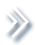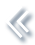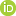####ArchivesReview Articles

## Achieving the oracle property of OEM with nonconvex penalties

Shifeng Xiong ,

Academy of Mathematics and Systems Science, Chinese Academy of Sciences, Beijing, ChinaBin Dai ,

Tower Research Capital, New York, NY, USAPeter Z. G. Qianpeterq@stat.wisc.edu

Pages 28-26 | Received 07 Mar. 2017, Accepted 30 Apr. 2017, Published online: 19 May. 2017,
• Abstract
• References
• Citations

Thepenalised least square estimator of non-convex penalties such as the smoothly clipped absolute deviation (SCAD) and the minimax concave penalty (MCP) is highly nonlinear and has many local optima. Finding a local solution to achieve the so-called oracle property is a challenging problem. We show that the orthogonalising EM (OEM) algorithm can indeed find such a local solution with the oracle property under some regularity conditions for a moderate but diverging number of variables.

• Bai, Z. D., & Yin, Y. Q. (1993). Limit of smallest eigenvalue of a large dimensional sample covariance matrix. Annals of Probability, 21, 12751294[Google Scholar]
• Breiman, L. (1995). Better subset regression using the nonnegative garrote. Technometrics, 37, 373384[Taylor & Francis Online]
• Breheny, P., & Huang, J. (2011). Coordinate descent algorithms for nonconvex penalized regression, with applications to biological feature selection. Annals of Applied Statistics, 5, 232253[Google Scholar]
• Bühlmann, P., & van de Geer, S. (2011). Statistics for high-dimensional data: Methods, theory and applications. Berlin: Springer[Google Scholar]
• Eicker, F. R. (1963). Asymptotic normality and consistency of the least squares estimators for families of linear regressions. The Annals of Mathematical Statistics, 34, 447456[Google Scholar]
• Fan, J., & Li, R. (2001). Variable selection via nonconcave penalized likelihood and its oracle properties. Journal of the American Statistical Association, 96, 13481360[Taylor & Francis Online]
• Fan, J., & Lv, J. (2008). Sure independence screening for ultrahigh dimensional feature space (with discussion). Journal of the Royal Statistical Society: Series B, 70, 849911[Google Scholar]
• Fan, J., & Lv, J. (2011). Properties of non-concave penalized likelihood with NP-dimensionality. IEEE Transactions on Information Theory, 57, 54675484[Google Scholar]
• Fan, J., & Peng, H. (2004). Non-concave penalized likelihood with diverging number of parameters. Annals of Statistics, 32, 928961[Google Scholar]
• Fan, J., Xue, L., & Zou, H. (2014). Strong oracle optimality of folded concave penalized estimation. Annals of Statistics, 42, 819849[Google Scholar]
• Fan, Y., & Tang, C. Y. (2013). Tuning parameter selection in high dimensional penalized likelihood. Journal of the Royal Statistical Society, Series B, 75, 531552[Google Scholar]
• Hoerl, A. E., & Kennard, R. W. (1970). Ridge regression: Biased estimation for nonorthogonal problems. Technometrics, 12, 5567[Taylor & Francis Online]
• Hsu, D., Kakade, S. M., & Zhang, T. (2012). A tail inequality for quadratic forms of sub-Gaussian random vectors. Electronic Journal of Probability, 17, 16[Google Scholar]
• Hunter, D. R., & Li, R. (2005). Variable selection using MM algorithms. Annals of Statistics, 33, 16171642[Google Scholar]
• Huo, X., & Chen, J. (2010). Complexity of penalized likelihood estimation. Journal of Statistical Computation and Simulation, 80, 747759[Taylor & Francis Online]
• Huo, X., & Ni, X. L. (2007). When do stepwise algorithms meet subset selection criteria? Annals of Statistics, 35, 870887[Google Scholar]
• Kim, Y., Choi, H., & Oh, H.-S. (2008). Smoothly clipped absolute deviation on high dimensions. Journal of American Statistical Association, 103, 16651673[Taylor & Francis Online]
• Lai, T. L., & Wei, C. Z. (1984). Moment inequalities with applications to regression and time series models. Inequalities in Statistics and Probability, IMS Lecture Notes-Monograph Series, 5, 165172[Google Scholar]
• Lanczos, C. (1950). An iteration method for the solution of the eigenvalue problem of linear differential and integral operators. Journal of Research of the National Bureau of Standards, 45, 255282[Google Scholar]
• Loh, P. L., & Wainwright, M. J. (2013). Regularized M-estimators with nonconvexity: Statistical and algorithmic theory for local optima. Advances in Neural Information Processing Systems, 26, 476484[Google Scholar]
• Mazumder, R., Friedman, J., & Hastie, T. (2011). SparseNet: Coordinate descent with non-convex penalties. Journal of American Statistical Association, 106, 11251138[Taylor & Francis Online]
• Meng, X. L. (2008). Discussion on one-step sparse estimates in nonconcave penalized likelihood models. Annals of Statistics, 36, 15421552[Google Scholar]
• Schifano, E. D., Strawderman, R., & Wells, M. T. (2010). Majorization–minimization algorithms for nonsmoothly penalized objective functions. Electronic Journal of Statistics, 4, 12581299[Google Scholar]
• Tibshirani, R. (1996). Regression shrinkage and selection via the lasso. Journal of the Royal Statistical Society: Series B, 58, 267288[Crossref]
• Tseng, P. (2001). Convergence of a block coordinate descent method for nondifferentiable minimization. Journal of Optimization Theory and Applications, 109, 475494[Google Scholar]
• Tseng, P., & Yun, S. (2009). A coordinate gradient descent method for nonsmooth separable minimization. Programs in Mathematics, 117, 387423[Google Scholar]
• Wang, H., Li, B., & Leng, C. (2009). Shrinkage tuning parameter selection with a diverging number of parameters. Journal of the Royal Statistical Society: Series B, 71, 671683[Google Scholar]
• Wang, H., Li, R., & Tsai, C-L. (2007). Tuning parameter selectors for the smoothly clipped absolute deviation method. Biometrika, 94, 553568[Google Scholar]
• Wang, L., Kim, Y., & Li, R. (2013). Calibrating nonconvex penalized regression in ultra-high dimension. Annals of Statistics, 41, 25052536[Google Scholar]
• Wang, S., & Jia, Z. (1994). Inequalities in matrix theory (In Chinese). Hefei: Anhui Education Press[Google Scholar]
• Xiong, S., Dai, B., Huling, J., & Qian, P. (2016). Orthogonalizing EM: A design-based least squares algorithm. Technometrics, 58, 285293[Taylor & Francis Online]
• Xiong, S., Dai, B., & Qian, P. (2011). OEM algorithm for least squares problems (Unpublished report). Retrieved from http://arxiv.org/abs/1108.0185 [Google Scholar]
• Zhang, C.-H. (2010). Nearly unbiased variable selection under minimax concave penalty. Annals of Statistics, 38, 894942[Google Scholar]
• Zou, H. (2006). The adaptive lasso and its oracle properties. Journal of the American Statistical Association, 101, 14181429[Taylor & Francis Online]
• Zou, H., & Li, R. (2008). One-step sparse estimates in nonconcave penalized likelihood models. Annals of Statistics, 36, 15091533[Google Scholar]